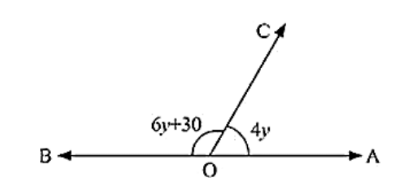# What value of y would make AOB a line in the below figure,

Question:

What value of y would make AOB a line in the below figure, If ∠AOB = 4y and ∠BOC = (6y + 30)?Solution:

Since, ∠AOC and ∠BOC are linear pairs

∠AOC + ∠BOC = 180°

6y + 30 + 4y = 180

10y + 30 = 180

10y = 180 - 30

10y = 150

y = 150/10

y = 15

Hence value of y that will make AOB a line is 15°